PSABM6WF - Student Facing Task---Acc7.5 Lesson 14 Solving Systems of Equations (8.EE.C.8 8.EE.C.8.a)
Part A)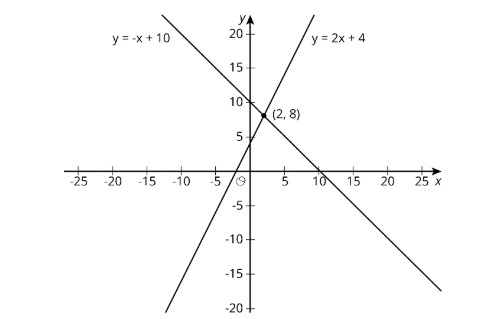Use the lines to decide whether each statement is true or false. Be prepared to explain your reasoning using the lines.

A solution to 8 = -x + 10 is 2.

Select one:
Part B)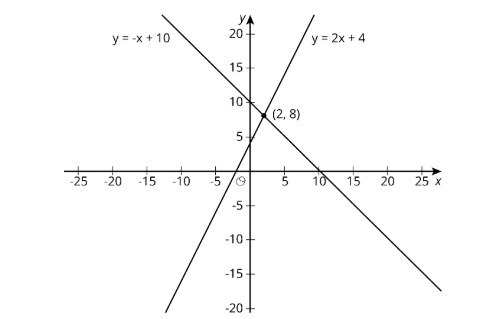Use the lines to decide whether each statement is true or false. Be prepared to explain your reasoning using the lines.

A solution to 8 = -x + 10 is 2.

Part C)Use the lines to decide whether each statement is true or false. Be prepared to explain your reasoning using the lines.

A solution to 2 = 2x + 4 is 8.

Select one:
Part D)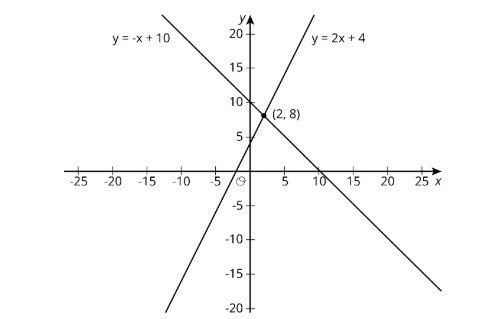Use the lines to decide whether each statement is true or false. Be prepared to explain your reasoning using the lines.

A solution to 2 = 2x + 4 is 8.

Part E)Use the lines to decide whether each statement is true or false. Be prepared to explain your reasoning using the lines.

A solution to -x + 10 = 2x + 4 is 8.

Select one:
Part F)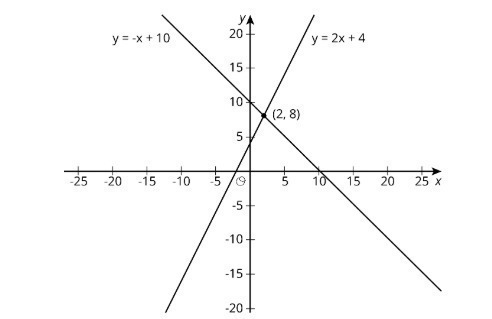Use the lines to decide whether each statement is true or false. Be prepared to explain your reasoning using the lines.

A solution to -x + 10 = 2x + 4 is 8.

Part G)Use the lines to decide whether each statement is true or false. Be prepared to explain your reasoning using the lines.

A solution to -x + 10 = 2x + 4 is 2.

Select one:
Part H)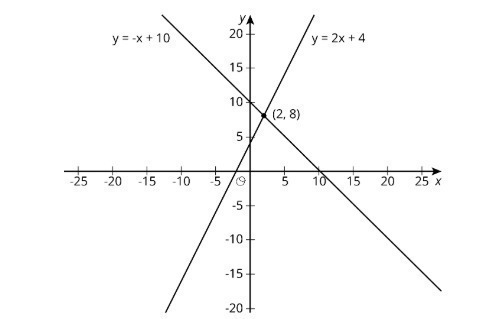Use the lines to decide whether each statement is true or false. Be prepared to explain your reasoning using the lines.

A solution to -x + 10 = 2x + 4 is 2.

Part I)Use the lines to decide whether each statement is true or false. Be prepared to explain your reasoning using the lines.

There are no values of x and y that make y = -x + 10 and y = 2x + 4 true at the same time.

Select one:
Part J)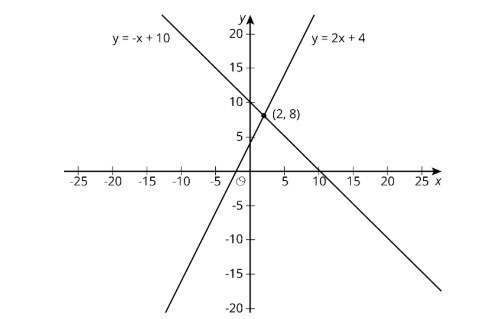Use the lines to decide whether each statement is true or false. Be prepared to explain your reasoning using the lines.

There are no values of x and y that make y = -x + 10 and y = 2x + 4 true at the same time.

Part A)

Here are three systems of equations graphed on a coordinate plane: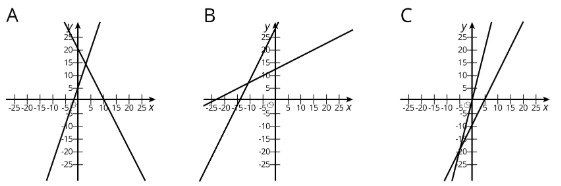Match each figure to one of the systems of equations shown here.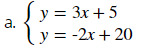Select one:
Part B)

Match each figure to one of the systems of equations shown here.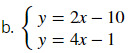Select one:
Part C)

Match each figure to one of the systems of equations shown here.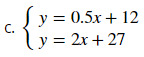Select one:
Part D)

Find the solution to each system and check that your solution is reasonable

based on the graph.

Systems of Equations A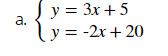(x,y)

with no spaces.

Part E)

Systems of Equation B.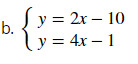(x,y)

with no spaces.

Part F)

Systems of Equations C: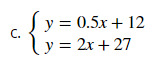(x,y)

with no spaces.

Part A)

Describe what the graph of a system of equations looks like when it has....

1 solution

Part B)

Describe what the graph of a system of equations looks like when it has....

0 solutions

Part C)

Describe what the graph of a system of equations looks like when it has....

infinitely many solutions

Part A)

The graphs of the equationsandintersect at (2,1).

Find A and B.

A = ____

Type your answer below as a number (example: 5, 3.1, 4 1/2, or 3/2):
Part B)

The graphs of the equationsandintersect at (2,1). Find A and B